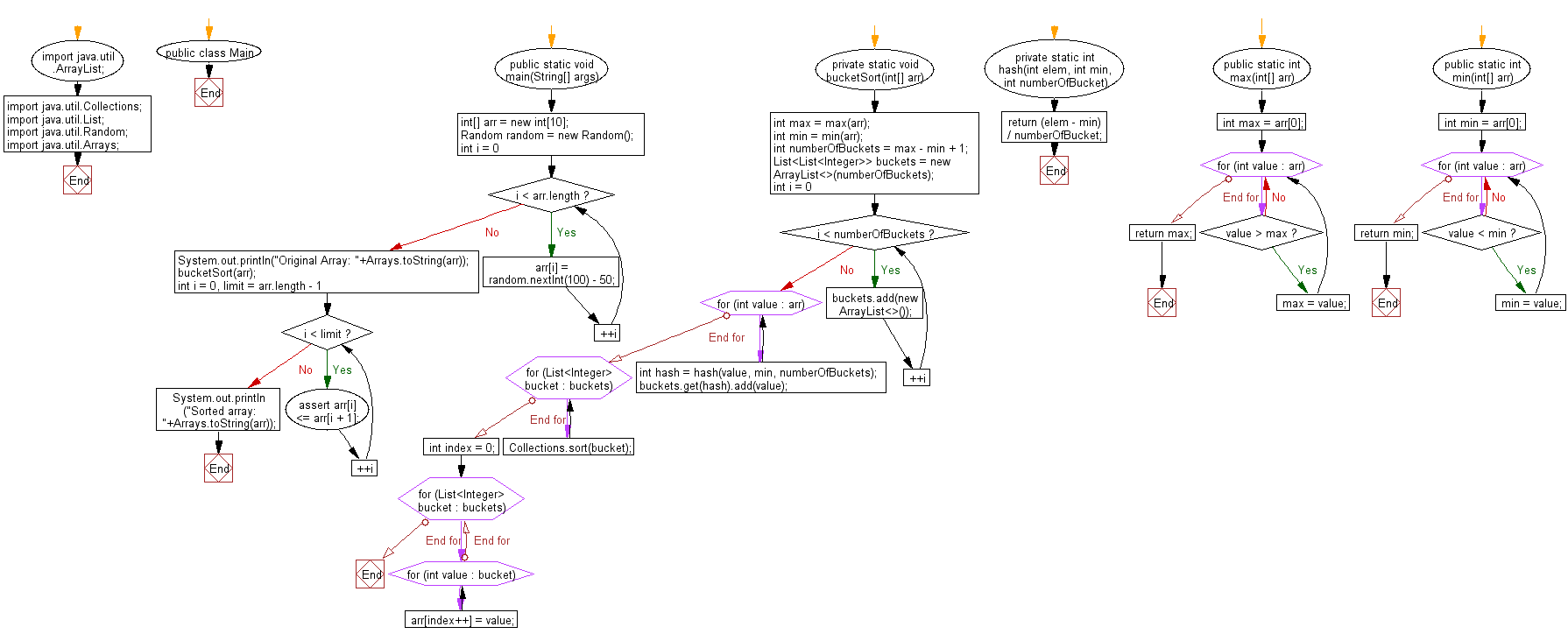﻿ Java exercises: Sort an array of given integers using Bucket Sort Algorithm - w3resource# Java Exercises: Bucket Sort Algorithm

## Java Sorting Algorithm: Exercise-19 with Solution

Write a Java program to sort an array of given integers using Bucket Sort Algorithm.

Bucket sort is a sorting algorithm that works by distributing the elements of an array into a number of buckets. Each bucket is then sorted individually, either using a different sorting algorithm, or by recursively applying the bucket sorting algorithm. It is a distribution sort, a generalization of pigeonhole sort, and is a cousin of radix sort in the most-to-least significant digit flavor.

Sample Solution:

Java Code:

``````import java.util.ArrayList;
import java.util.Collections;
import java.util.List;
import java.util.Random;
import java.util.Arrays;
/*Ref. https://bit.ly/3mAyUj3 */
/** Wikipedia: https://en.wikipedia.org/wiki/Bucket_sort */
public class Main {
public static void main(String[] args) {
int[] arr = new int;
/* generate 10 random numbers from -50 to 49 */
Random random = new Random();
for (int i = 0; i < arr.length; ++i) {
arr[i] = random.nextInt(100) - 50;
}
System.out.println("Original Array: "+Arrays.toString(arr));
bucketSort(arr);
/* check array is sorted or not */
for (int i = 0, limit = arr.length - 1; i < limit; ++i) {
assert arr[i] <= arr[i + 1];
}
System.out.println("Sorted array: "+Arrays.toString(arr));
}
/**
* BucketSort algorithms implements
*
* @param arr the array contains elements
*/
private static void bucketSort(int[] arr) {
/* get max value of arr */
int max = max(arr);
/* get min value of arr */
int min = min(arr);
/* number of buckets */
int numberOfBuckets = max - min + 1;
List<List<Integer>> buckets = new ArrayList<>(numberOfBuckets);
/* init buckets */
for (int i = 0; i < numberOfBuckets; ++i) {
}
/* store elements to buckets */
for (int value : arr) {
int hash = hash(value, min, numberOfBuckets);
}
/* sort individual bucket */
for (List<Integer> bucket : buckets) {
Collections.sort(bucket);
}
/* concatenate buckets to origin array */
int index = 0;
for (List<Integer> bucket : buckets) {
for (int value : bucket) {
arr[index++] = value;
}
}
}
/**
* Get index of bucket which of our elements gets placed into it.
*
* @param elem the element of array to be sorted
* @param min min value of array
* @param numberOfBucket the number of bucket
* @return index of bucket
*/
private static int hash(int elem, int min, int numberOfBucket) {
return (elem - min) / numberOfBucket;
}
/**
* Calculate max value of array
*
* @param arr the array contains elements
* @return max value of given array
*/
public static int max(int[] arr) {
int max = arr;
for (int value : arr) {
if (value > max) {
max = value;
}
}
return max;
}
/**
* Calculate min value of array
*
* @param arr the array contains elements
* @return min value of given array
*/
public static int min(int[] arr) {
int min = arr;
for (int value : arr) {
if (value < min) {
min = value;
}
}
return min;
}
}
```
```

Sample Output:

```Original Array: [14, -15, 15, 17, 32, -4, 33, -42, 38, -36]
Sorted array: [-42, -36, -15, -4, 14, 15, 17, 32, 33, 38]
```

Flowchart:Java Code Editor:

What is the difficulty level of this exercise?

﻿

## Java: Tips of the Day

Array vs ArrayLists:

The main difference between these two is that an Array is of fixed size so once you have created an Array you cannot change it but the ArrayList is not of fixed size. You can create instances of ArrayLists without specifying its size. So if you create such instances of an ArrayList without specifying its size Java will create an instance of an ArrayList of default size.

Once an ArrayList is full it re-sizes itself. In fact, an ArrayList is internally supported by an array. So when an ArrayList is resized it will slow down its performance a bit as the contents of the old Array must be copied to a new Array.

At the same time, it's compulsory to specify the size of an Array directly or indirectly while creating it. And also Arrays can store both primitives and objects while ArrayLists only can store objects.

Ref: https://bit.ly/3o8L2KH